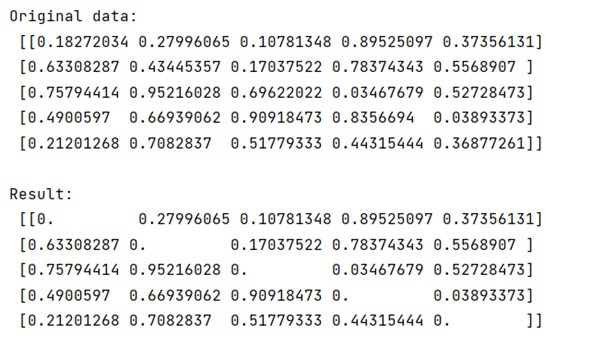# Change the values of the diagonal of a NumPy matrix

Learn, how to change the values of the diagonal of a NumPy matrix in Python?
Submitted by Pranit Sharma, on March 20, 2023

## Changing the values of the matrix's diagonal

Diagonals of an array are defined as a set of those points where the row and the column coordinates are the same.

To change the values of a diagonal of a matrix, we can use numpy.diag_indices_from() to get the indices of the diagonal elements of your array. Then set the value of those indices, we can simply assign them any value we want.

Let us understand with the help of an example,

## Python code to change the values of the diagonal of a NumPy matrix

```# Import numpy
import numpy as np

# Creating a numpy array
arr = np.random.rand(5, 5)

# Display original data
print("Original data:\n",arr,"\n")

# Getting diagonal indices
ind = np.diag_indices_from(arr)

# Giving the diagonals some value
arr[ind] = 0

# Display result
print("Result:\n",arr,"\n")
```

### Output